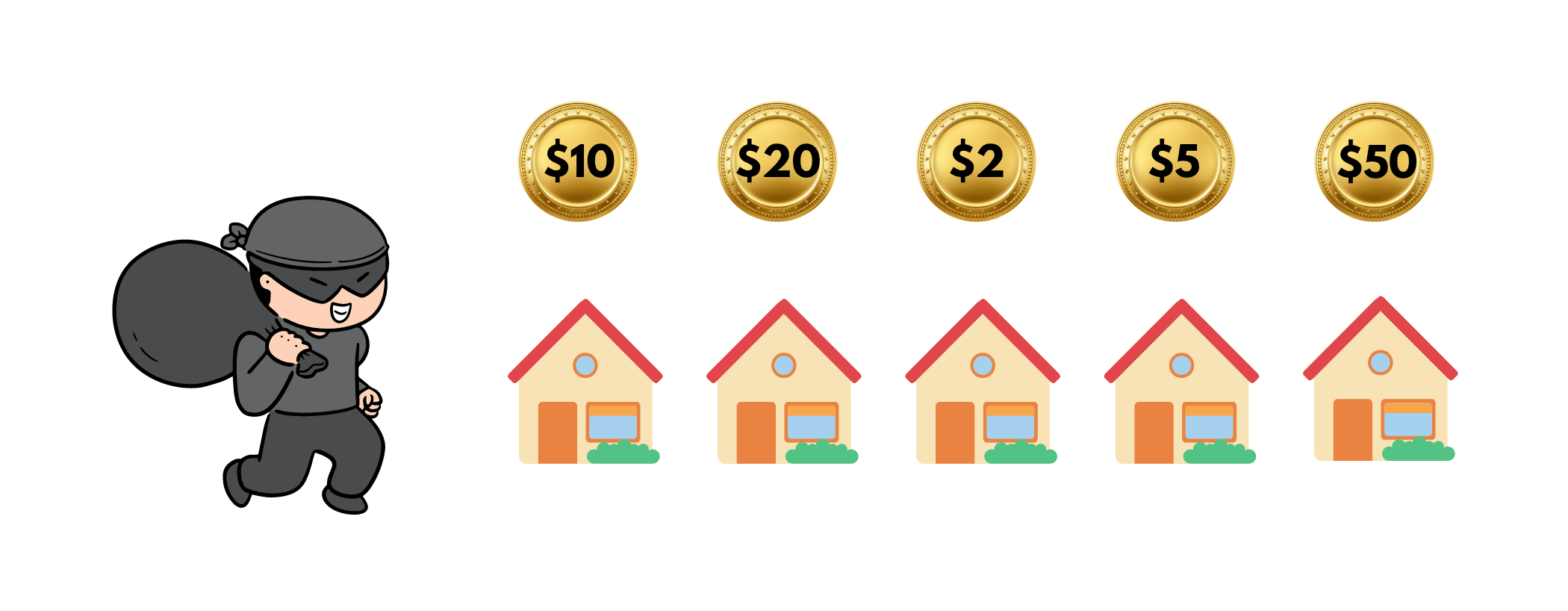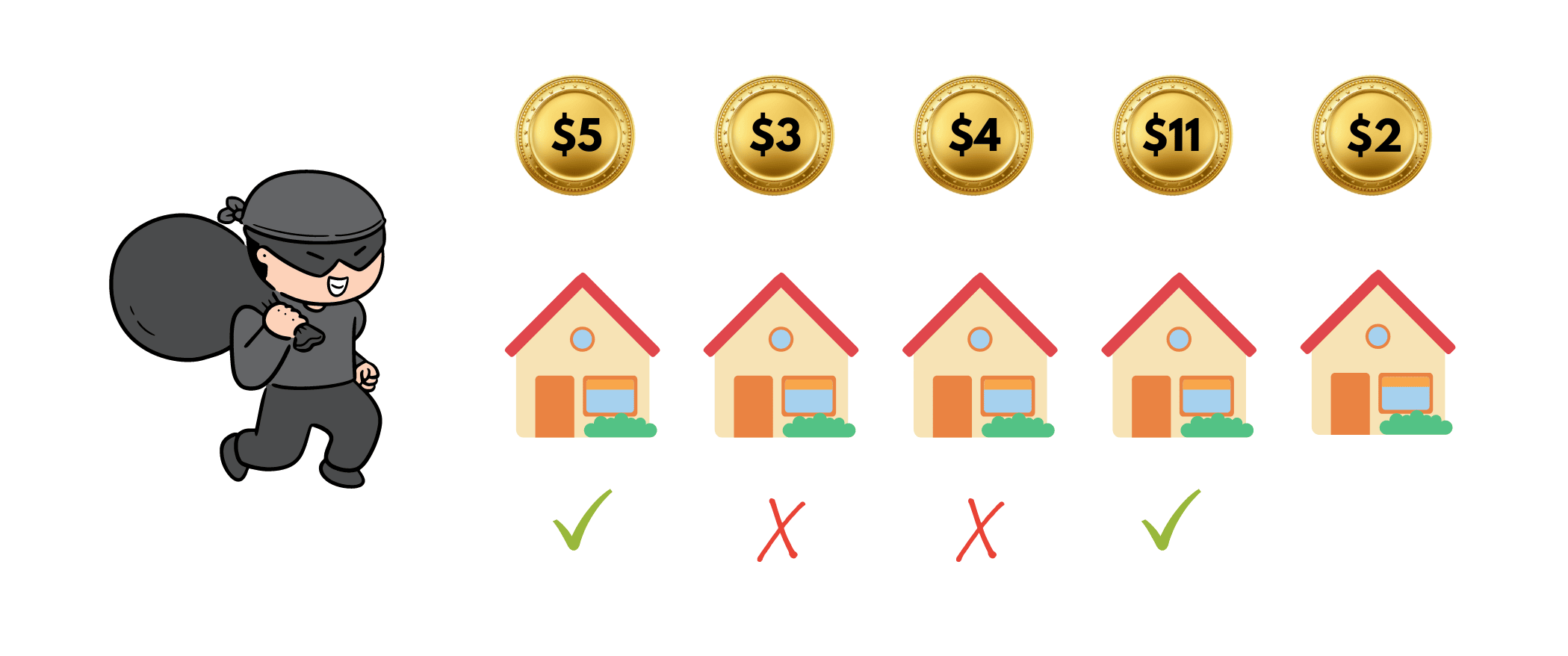# House Robber Problem Algorithm & Solution (C++ & Java)

In today's scenario with thousands of students preparing for interviews for MAANG and other remote jobs, acing at DSA and working hard on platforms like LeetCode and CodeChef has become the trend. So, we try to bring forward to you the most important questions for your preparation.

So, in today's tech blog we'll be discussing yet another dynamic programming problem that has gained quite a popularity, called House Robber Problem. The idea is to find the maximum possible stolen value from houses. We will also provide the source codes in C++ and Java languages.

## House Robber Problem Statement

Here is the problem statement for the House Robber Problem: You are given an array of 'n' houses in a lane. Each house contains some amount of money depicted by the value of the array element. There is a robber who wants to rob the houses in such a way as to get the maximum loot money from the houses.

Now, the robber cannot steal from two adjacent houses. You have to find the maximum possible amount of money the robber can steal from the houses in the given lane. You can also take a look at the below image.Let's take a look at an example:

Input: [5,3,4,11,2]

Output: 16

Explanation: The robber will steal from the 1st and 4th house, that is, 5 + 11 = 16. Take a look at the given image.Why? Well, when he comes across the first house, he'll loot it as the value of the house next to the first one is less. Later, he cannot steal money from the 2nd house so he moves to the third one. But, if he steals the money from the third house, he'll miss the opportunity to steal an even larger amount from the fourth house.

So, he steals the third house and steals from the fourth one. Thereafter, he cannot steal from the last one. Thus, the total amount comes out to be 16.

## Intuition behind solving the question

After reading the above explanation of the given example, I'm sure you must have gained a basic understanding of the problem. So, let's see how you need to go through this problem. Getting an optimized solution is a whole other thing which is explained in the next portion, but getting the intuition to move forward is important. So, read on!

Now, you are given an array and the robber has to take out the maximum value from the houses. But, he cannot steal from two adjacent houses. Understand it like this, if I put both my hands with candies in them, and I have to choose only one hand's candies. Which one will you pick? Obviously, the one with more! The same is with this robber.

Now, we'll start from the beginning of the array. If the money in the first house is greater than the second, the robber will steal from the first one, or else the second one. Now, you need to remember that if he steals from the second one, he cannot steal from the third one.

So, again monitoring the values of the house money is the crucial part of this question. Below, we have given certain types of solutions for you to get answers to the problem.

## House Robber Problem Solutions

Here are the 4 best solutions for this problem:

### Method 01) Naive Approach using Recursion

The easiest way to solve this question is using recursion. Here, we've provided the algorithm:

```->Input array as money in houses from the user.
-> Find the size of array.
-> Create a recursive function with base case n<0.
-> Choose the current element if previous one is not chosen.
-> Skip the current element if previous one is already chosen.```

Time Complexity for this method is  O(2n) and Space Complexity is O(1).

### Method 02) Top-Down Approach in Dynamic Programming

Although the recursive solution was good, dynamic programming has its own advantages. Here is the algorithm:

```-> Create a DP vector of size n+1 and value -1 and variables pick and not pick.
-> Create a recursive function
-If n < 0 possible stolen value is 0.
-If n = 0 possible stolen value is hval.
-> If DP[n] != -1 possible stolen value is DP[n].
-> Set pick as pick = hval[n] +  maxLoot(hval, n-2, DP)
-> Set notpick as notPick = maxLoot(hval, n-1, DP).
-> Set Dp[n] = max(pick, notPick) and return DP[n]```

Time Complexity for this method is  O(2n) and Space Complexity is O(2n).

### Method 03) Bottom-Up Approach

Even in dynamic programming, we have more than two methods for you to solve this question. So, read this algorithm to understand the following method:

```-> Create an extra space DP array to store the sub-problems.
-> Tackle the following basic cases,
-If the length of the array is 0, print 0.
-If the length of the array is 1, print the first element.
-If the length of the array is 2, print a maximum of two elements.
-> Update dp as array and dp as maximum of array and array
-> Traverse the array from the second element (2nd index) to the end of the array.
-> For every index, update dp[i] as a maximum of dp[i-2] + array[i] and dp[i-1], this step defines the two cases if an element is selected then the previous element cannot be selected and if an element is not selected then the previous element can be selected.
-> Print the value dp[n-1]
```

Time Complexity for this method is  O(n) and Space Complexity is O(n).

### Method 04) Constant Space Method

Since space complexity in the previous methods was quite high, in this method we're trying to remove the extra space used by using the constant space method in Dynamic Programming. Let's take a look at the algorithm:

```-> Tackle some basic cases,
-if the length of the array is 0, print 0,
-if the length of the array is 1, print the first element,
-if the length of the array is 2, print a maximum of two elements.
-> Create two variables value1 and value2, value1 as array and value2 as maximum of array and array and a variable max_val to store the answer
-> Traverse the array from the second element (2nd index) to the end of the array.
-> For every index, update max_val as the maximum of value1 + array[i] and value2, this step defines the two cases, if an element is selected then the previous element cannot be selected and if an element is not selected then the previous element can be selected.
-> For every index, Update value1 = value2 and value2 = max_val
-> Print the value of max_val
```

Time Complexity for this method is O(n) and Space Complexity is O(1).

## Code Implementation in C++

Here is the C++ implementation for House Robber Problem:

```#include
using namespace std;
int maxAmount(int* arr, int n)
{
if (n == 0)
return 0;

int v1 = arr;
if (n == 1)
return v1;

int v2 = max(arr, arr);
if (n == 2)
return v2;
int max_val;
for (int i = 2; i < n; i++) {
max_val = max(arr[i] + v1, v2);
v1 = v2;
v2 = max_val;
}

return max_val;
}
int main()
{
int arr[] = { 6, 7, 1, 3, 8, 2, 4 };
int n = sizeof(arr) / sizeof(arr);
cout << "Maximum loot possible : " << maxAmount(arr, n);
return 0;
}
```

## Code Implementation in Java

Here is the Java implementation for House Robber Problem:

```import java.io.*;
class GFG {
static int maxAmount(int arr[], int n)
{
if (n == 0)
return 0;

int v1 = arr;
if (n == 1)
return v1;

int v2 = Math.max(arr, arr);
if (n == 2)
return v2;
int max_val = 0;
for (int i = 2; i < n; i++) {
max_val = Math.max(arr[i] + v1, v2);
v1 = v2;
v2 = max_val;
}
return max_val;
}
public static void main(String[] args)
{
int arr[] = { 6, 7, 1, 3, 8, 2, 4 };
int n = arr.length;
System.out.println("Maximum loot possible : "
+ maxAmount(arr, n));
}
}
```

Output:

```Maximum loot possible : 19
```

## Final Words

So, in this tech blog, we have understood the recursive as well as three ways of solving this problem using the famous dynamic programming concept. Although the working of a recursive solution is just fine, we all know that decreasing space complexity is quite necessary, so using the last method works the best.

### FavTutor - 24x7 Live Coding Help from Expert Tutors!